# The QUANTLIFE Procedure

### Quantile Regression

Suppose that
is a dependent variable of interest (such as the survival time or some monotone transformation of the survival time) and x is a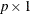vector of covariates. The observed data consist of a random sample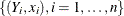from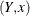.

You can use regression analysis to explore the relationship between the responseand its predictor x. Classical linear regression estimates the conditional mean function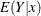by using a linear predictor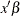; a linear quantile regression estimates theth conditional quantile function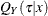by using a different linear predictor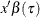, where the quantile levelranges between 0 and 1. For example,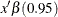is the linear predictor for the 0.95th quantile (commonly referred to as the 95th percentile).

The quantile regression coefficient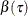can be estimated by minimizing the following objective function over b: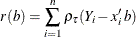The loss function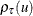is defined as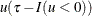, in contrast to the square loss function for classical linear regression.

When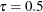, the coefficient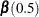minimizes the sum of absolute residuals, which corresponds to median regression (or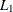regression).

The following set of regression quantiles is referred to as the quantile process, and it completely describes the conditional distribution of, given the predictor x: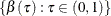When all the observations are observed, you can use the QUANTREG procedure to estimate the quantile functionand draw statistical inference about the regression parameters. For more information, see Chapter 83: The QUANTREG Procedure.

However, when the observations are incomplete, as is the case with censored data in survival analysis, the classical quantile regression method is not appropriate. The QUANTLIFE procedure implements appropriate quantile regression methods to model the relationship between the responseand the predictor x.